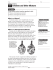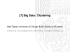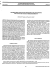# Poster - University of Sussex

## Transcription

Poster - University of Sussex
```Convex Relaxation of Mixture Regression with Efficient Algorithms
Authors
1
1
1
2
Novi Quadrianto | Tibério S. Caetano | John Lim | Dale Schuurmans
1: NICTA & Australian National University | 2: University of Alberta
Abstract
• We develop a convex relaxation of maximum a posteriori estimation of a mixture of regression model.
Semidefinite Relaxation
Problem (1) can be relaxed to
• We reformulate the relaxation problem to eliminate the need for general semidefinite programming.
• We provide two reformulations that admit fast algorithms. The first is a max-min spectral reformulation
exploiting quasi-Newton descent. The second is a min-min reformulation consisting of fast alternating
• We provide experiments in a real problem of motion segmentation from video data.
Applications
Toy Data
"
#
T
1 2 T
y
1 y
T
min
max − σ c c −
− c M XX
−c .
2
2
2
2α σ
M:tr M=t,γtI<M<0 c
σ
(2)
(Sketchy) Steps
• Dataset: 30 synthetic data points is generated according to yi = (πi ⊗ xi)w + i, with xi ∈ R, i ∼ N(0, 1)
and w ∈ U(0, 1). The response variable yi is assumed to be generated from a mixture of 5 components .
• Performance comparison with EM (100 random restarts was used to avoid poor local optima).
1 wT ΨT Ψw).
• Compute convex conjugate of the log-partition function (i.e. 2σ
2
• Error rates are 0.347 ± 0.086 (EM) and 0.280 ± 0.063 (Max-Min) across 10 different runs.
• Relax the constraints on the assignment matrix
Mixture of Regression Problem
⊆
{ΠΠT : Π ∈ {0, 1}t×k , Π1 = 1, max(diag(ΠT Π)) ≤ γt}
{M : M ∈ Rt×t , tr M = t, γtI < M < 0}.
Note : The relaxation of Problem (1) to (2) only refers to loosening the set of feasible solutions and no
relaxation has been introduced in the objective function. However, solving (2) directly is prohibitive for
medium to large scale problems as it requires a general semidefinite solver.
Algorithm 1: Max-Min Reformulation
Ground Truth
Max-Min
EM
Motion Segmentation from Video Data
The ”hammer” (Overton & Womersley 1993)
Let V ∈ Rt×t , V = V T and its EVD, PT V P = Λ((λ1, . . . , λt )), then
max
Linear regression (left) and mixture of regression (right); Gaffney & Smyth 1999
Given
• a labeled training set D comprising t input-output pair {(x1, y1), . . . , (xt , yt )};
• assumption that the output variable y is generated by a mixture of k components;
• no information about which component of the mixture generates each output variable yi.
M:tr(M)=q,I<M<0
tr MV T =
q
X
i=1
λi
and
argmax
M:tr(M)=q,I<M<0
• Dataset: Hopkins 155 (http://www.vision.jhu.edu/data/hopkins155/).
tr MV T 3 Pq PTq .
• Given a pair of corresponding points pi and qi from two frames and k motion groups, we have the following
Pk
T
epipolar equation ( Li 2007), qi ( j=1 πi j F j)pi = 0.
Pk
y x π
π
π
x
x
T
T
x
• Redefine [qi pi qi pi qi pi .... qi pi ] := xi and vec(F j ) := w, our problem is now j=1 πi j xiT w j = 0.
To use the ”hammer”, Problem (2) is reformulated to


 1 2 T

t
T
T
max − σ c c −
max
tr( M̄(XX (ȳ − c)(ȳ − c) )) .
c
2
2αq M̄:tr M̄=q,I< M̄<0
Find
• a regression model f : X → Y for each of the mixture component.
The Model
(Sketchy) Steps
• Simply by interchanging min M and maxc, invoking distributivity property, rearranging the terms and definy
ing ȳ := σ2 , problem (2) can be rewritten as
"
1
1 2 T
max
max − σ c c −
c
2
2α M:tr M=t,γtI<M<0
Denote
• X ∈ Rt×n as a matrix of input and y ∈ Rt×1 as a vector of output variables;
• Π ∈ {0, 1}t×k , Π1 = 1 and max(diag(ΠT Π)) ≤ γt (bounding the size of the largest component) as the hidden
assignment matrix.
Assume
• a linear regression model yi|xi, πi = ψiw + i,
i ∼ N(0, σ2) w.r.t a feature representation ψi = πi ⊗ xi.
Gaussian Likelihood
With the Gaussian noise model , our likelihood is then
#
"
1
1
p(yi|xi, πi; w) = √
exp − 2 (ψiw − yi)2 .
2σ
2πσ2
Log-Posterior Optimization
With an additional assumption of Gaussian prior on the parameter w, the minimization of (negative) logposterior is now in the form of
"
#
1 T T
1 T
α T
min
w Ψ Ψw − 2 y Ψw + w w ,
(1)
2
2
Π,w 2σ
σ
s.t. constraints on the assignment matrix Π.
#
tr(M(XX T (ȳ − c)(ȳ − c)T )) .
• Let q = {u : u = max{1, . . . , t}, u ≤ γ−1} and define M̄ := (q/t)M to transform the constraint set from
{M : tr M = t, γtI < M < 0} to {M : tr M = q, I < M < 0}.
Algorithm 2: Min-Min Reformulation
Problem (2) is also equivalent to
"
#
1 T
1
α
T
T
T
T
T M −1 A) .
min
min
y
diag(XA
)
+
diag(XA
)
diag(XA
)
+
tr(A
2γt
{M:IM0,tr M=1/γ} A
σ2
2σ2
σ2 T
γt
Steps; (2) =
min
max
− c c−
tr(C T MC)
2
2α
{M:IM0,tr M=1/γ} {c,C:C=Λ(c−ȳ)X}
σ2 T
γt
=
min
min max − c c −
tr(C T MC) + tr(AT C) − tr(AT Λ(c − ȳ)X).
2
2α
{M:IM0,tr M=1/γ} A c,C
α M −1 A.
Lastly, c and C can be solved as c = − σ12 diag(XAT ) and C = γt
Ground Truth
EM
Max-Min
Min-Min
References
• S. Gaffney and P. Smyth. Trajectory clustering with mixtures of regression models. In ACM SIGKDD,
volume 62, pages 63–72, 1999.
• M. Overton and R. Womersley. Optimality conditions and duality theory for minimizing sums of the
largest eigenvalues of symmetric matrices. Mathematical Programming, 62:321–357, 1993.
• H. Li. Two-view motion segmentation from linear programming relaxation. In CVPR, 2007.
```

### LRTrek - ActFX Algorithmic Trading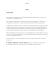### Pastel de choclo - corn cake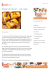### Milk Barfi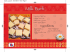### termoplast plus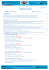### Changes in Matter - Pearson SuccessNet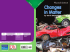### A GUIDE TO DETERMINING SAMPLE SIZE A PRIORI INTRODUCTION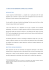### Guidelines for grant of permission to travel by Airlines other than Air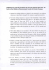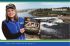### Multiple Linear Regression Objective: 1/9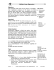### MesoDermal Mesotherapy Cocktails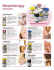### Cherry-Cabernet Beef Tournedos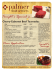### How to write an article Pieter M. Kroonenberg, Contents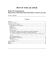### Stepping Stones March 2014 Newsletter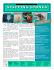### 1. Ho: µ= 28 years vs. Ha: µ &gt; 28 years.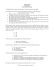### 8. The Simple Linear Regression Model 8.1 The “True” Model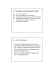### texas calculatem key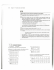### CHAPTER 6 COST ESTIMATION Jinashree Rajendrakumar Jisha Nambiar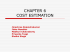### axis major reduced regression rma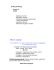### UNIVERSITY OF TORONTO AT SCARBOROUGH Sample Exam STAC67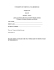### 1 Solutions and Other Mixtures